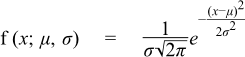#Boost C++ Libraries

...one of the most highly regarded and expertly designed C++ library projects in the world.

This is the documentation for an old version of Boost. Click here to view this page for the latest version.

#### Normal (Gaussian) Distribution

`#include <boost/math/distributions/normal.hpp>`
```namespace boost{ namespace math{

template <class RealType = double,
class Policy   = policies::policy<> >
class normal_distribution;

typedef normal_distribution<> normal;

template <class RealType, class Policy>
class normal_distribution
{
public:
typedef RealType value_type;
typedef Policy   policy_type;
// Construct:
normal_distribution(RealType mean = 0, RealType sd = 1);
// Accessors:
RealType mean()const; // location.
RealType standard_deviation()const; // scale.
// Synonyms, provided to allow generic use of find_location and find_scale.
RealType location()const;
RealType scale()const;
};

}} // namespaces
```

The normal distribution is probably the most well known statistical distribution: it is also known as the Gaussian Distribution. A normal distribution with mean zero and standard deviation one is known as the Standard Normal Distribution.

Given mean μ  and standard deviation σ  it has the PDF:The variation the PDF with its parameters is illustrated in the following graph:The cumulative distribution function is given byand illustrated by this graph##### Member Functions
```normal_distribution(RealType mean = 0, RealType sd = 1);
```

Constructs a normal distribution with mean mean and standard deviation sd.

Requires sd > 0, otherwise domain_error is called.

```RealType mean()const;
RealType location()const;
```

both return the mean of this distribution.

```RealType standard_deviation()const;
RealType scale()const;
```

both return the standard deviation of this distribution. (Redundant location and scale function are provided to match other similar distributions, allowing the functions find_location and find_scale to be used generically).

##### Non-member Accessors

All the usual non-member accessor functions that are generic to all distributions are supported: Cumulative Distribution Function, Probability Density Function, Quantile, Hazard Function, Cumulative Hazard Function, mean, median, mode, variance, standard deviation, skewness, kurtosis, kurtosis_excess, range and support.

The domain of the random variable is [-[max_value], +[min_value]]. However, the pdf of +∞ and -∞ = 0 is also supported, and cdf at -∞ = 0, cdf at +∞ = 1, and complement cdf -∞ = 1 and +∞ = 0, if RealType permits.

##### Accuracy

The normal distribution is implemented in terms of the error function, and as such should have very low error rates.

##### Implementation

In the following table m is the mean of the distribution, and s is its standard deviation.

Function

Implementation Notes

pdf

Using the relation: pdf = e-(x-m)2/(2s2) / (s * sqrt(2*pi))

cdf

Using the relation: p = 0.5 * erfc(-(x-m)/(s*sqrt(2)))

cdf complement

Using the relation: q = 0.5 * erfc((x-m)/(s*sqrt(2)))

quantile

Using the relation: x = m - s * sqrt(2) * erfc_inv(2*p)

quantile from the complement

Using the relation: x = m + s * sqrt(2) * erfc_inv(2*p)

mean and standard deviation

The same as `dist.mean()` and `dist.standard_deviation()`

mode

The same as the mean.

median

The same as the mean.

skewness

0

kurtosis

3

kurtosis excess

0

 Copyright © 2006-2010, 2012, 2013 Paul A. Bristow, Christopher Kormanyos, Hubert Holin, Bruno Lalande, John Maddock, Johan Råde, Gautam Sewani, Benjamin Sobotta, Thijs van den Berg, Daryle Walker and Xiaogang Zhang Distributed under the Boost Software License, Version 1.0. (See accompanying file LICENSE_1_0.txt or copy at http://www.boost.org/LICENSE_1_0.txt)